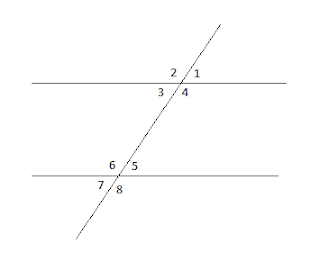### Common Errors in Secondary Mathematics

Common Errors Committed  by the  Students  in Secondary Mathematics   Errors  that students often make in doing secondary mathematics  during their practice and during the examinations  and their remedial measures are well explained here stp by step.  Some Common Errors in Mathematics

# Different Types of Angles

Beautiful and useful blog on different types of angles, useful for the students of 5th on-word . Complete knowledge about different types of angles in mathematics

## Different Types of Angles

Zero Angle:- Angle of 0o is called zero angle.

Acute angle :- Angle greater than 0o but less than 90o is called acute angle.

Right Angle:- Angle of 90o is called right angle.

Obtuse Angle:- Angle greater than 90o but less than 180o is called obtuse angle.
Straight Angle :- Angle of 180o is called straight angle.

Reflex angle :- Angle greater than 180o but less than 360o is called reflex angle.
Complete angle:- Angle of 360o is called complete angle.

Linear Pair of Angles:-
When sum of two adjacent angles is  180o, then pair of angles is called  a linear pair of angles .

Two angles with one common arm are called adjacent angles.

Complementary Angles :-
If sum of any two angles is 90o then angles are called complementary angles.

Supplementary Angles:-
If sum of any two angles is 180o then angles are called supplementary angles.

Vertically Opposite Angles:-
When two lines intersect each other then vertically opposite angles are formed. Vertically opposite angles are always equal.
Parallel Lines and Angles

When two or more parallel lines are intersected by a transversal then :-Alternate angles are equal:
Corresponding angles are equal:-
Alternate Exterior Angles are Equal:-
Co - interior Angles are Supplementary :-
Vertically Opposite angles are equal:-
Sum of three angles of a triangle is 180o
Exterior angle of a triangle is equal to the sum of interior opposite  angles.

🙏

1.2.Thanks